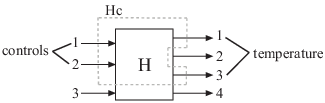### Specify Model Time Units

This example shows how to specify time units of a transfer function model.

The `TimeUnit` property of the `tf` model object specifies units of the time variable, time delays (for continuous-time models), and the sample time Ts (for discrete-time models). The default time units is `seconds`.

Create a SISO transfer function model $sys=\frac{4s+2}{{s}^{2}+3s+10}$ with time units in milliseconds:

```num = [4 2]; den = [1 3 10]; sys = tf(num,den,'TimeUnit','milliseconds');```

You can specify the time units of any dynamic system in a similar way.

The system time units appear on the time- and frequency-domain plots. For multiple systems with different time units, the units of the first system are used if the time and frequency units in the Toolbox Preferences Editor are `auto`.

Note

Changing the `TimeUnit` property changes the system behavior. If you want to use different time units without modifying system behavior, use `chgTimeUnit`.

### Interconnect Models with Different Time Units

This example shows how to interconnect transfer function models with different time units.

To interconnect models using arithmetic operations or interconnection commands, the time units of all models must match.

1. Create two transfer function models with time units of milliseconds and seconds, respectively.

```sys1 = tf([1 2],[1 2 3],'TimeUnit','milliseconds'); sys2 = tf([4 2],[1 3 10]); ```
2. Change the time units of `sys2` to milliseconds.

```sys2 = chgTimeUnit(sys2,'milliseconds'); ```
3. Connect the systems in parallel.

`sys = sys1+sys2;`

### Specify Frequency Units of Frequency-Response Data Model

This example shows how to specify units of the frequency points of a frequency-response data model.

The `FrequencyUnit` property specifies units of the frequency vector in the `Frequency` property of the `frd` model object. The default frequency units are `rad/TimeUnit`, where `TimeUnit` is the time unit specified in the `TimeUnit` property.

Create a random SISO frequency-response data model with frequency data in GHz.

```resp = randn(7,1) + i*randn(7,1); freq = logspace(-2,2,7); sys = frd(resp,freq,'FrequencyUnit','GHz');```

You can independently specify the units in which you measure the frequency points and sample time in the `FrequencyUnit` and `TimeUnit` properties, respectively. You can also specify the frequency units of a `genfrd` in a similar way.

The frequency units appear on the frequency-domain plots. For multiple systems with different frequency units, the units of the first system are used if the frequency units in the Toolbox Preferences Editor is `auto`.

Note

Changing the `FrequencyUnit` property changes the system behavior. If you want to use different frequency units without modifying system behavior, use `chgFreqUnit`.

### Extract Subsystems of Multi-Input, Multi-Output (MIMO) Models

This example shows how to extract subsystems of a MIMO model using MATLAB® indexing and using channel names.

Extracting subsystems is useful when, for example, you want to analyze a portion of a complex system.

Create a MIMO transfer function.

```G1 = tf(3,[1 10]); G2 = tf([1 2],[1 0]); G = [G1,G2]; ```

Extract the subsystem of `G` from the first input to all outputs.

`Gsub = G(:,1);`

This command uses MATLAB indexing to specify a subsystem as `G(out,in)`, where `out` specifies the output indices and `in` specifies the input indices.

Using channel names, you can use MATLAB indexing to extract all the dynamics relating to a particular channel. By using this approach, you can avoid having to keep track of channel order in a complex MIMO model.

Assign names to the model inputs.

```G.InputName = {'temperature';'pressure'}; ```

Because `G` has two inputs, use a cell array to specify the two channel names.

Extract the subsystem of `G` that contains all dynamics from the `'temperature'` input to all outputs.

`Gt = G(:,'temperature');`

`Gt` is the same subsystem as `Gsub`.

Note

When you extract a subsystem from a state-space (`ss`) model, the resulting state-space model may not be minimal. Use `sminreal` to eliminate unnecessary states in the subsystem.

### Specify and Select Input and Output Groups

This example shows how to specify groups of input and output channels in a model object and extract subsystems using the groups.

Input and output groups are useful for keeping track of inputs and outputs in complex MIMO models.

1. Create a state-space model with three inputs and four outputs.

`H = rss(3,4,3);`
2. Group the inputs as follows:

• Inputs 1 and 2 in a group named `controls`

• Outputs 1 and 3 to a group named `temperature`

• Outputs 1, 3, and 4 to a group named `measurements`

```H.InputGroup.controls = [1 2]; H.OutputGroup.temperature = [1 3]; H.OutputGroup.measurements = [1 3 4]; ```

`InputGroup` and `OutputGroup` are structures. The name of each field in the structure is the name of the input or output group. The value of each field is a vector that identifies the channels in that group.

3. Extract the subsystem corresponding to the `controls` inputs and the `temperature` outputs.

You can use group names to index into subsystems.

`Hc = H('temperature','controls')`

`Hc` is a two-input, two-output `ss` model containing the I/O channels from the `'controls'` input to the `'temperature'` outputs.

You can see the relationship between `H` and the subsystem `Hc` in this illustration.